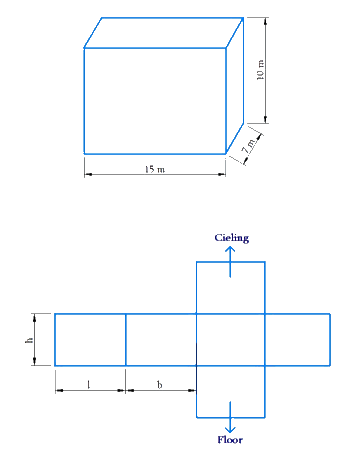# Ex.11.3 Q5 Mensuration Solution - NCERT Maths Class 8

## Question

Daniel is painting the walls and ceiling of a cuboidal hall with length, breadth and height of $$15\,\rm{ m}, 10\,\rm{ m}$$ and $$7\,\rm{ m}$$ respectively. From each can of paint $$100\,\rm{ m^2}$$ of area is painted. How many cans of paint will she need to paint the room?

Video Solution
Mensuration
Ex 11.3 | Question 5

## Text Solution

What is Known?

Shape of the hall and its length, breadth and height.

What is unknown?

Number of cans of paint required to paint the room.

Reasoning:The side of the hall makes the lateral surface area of cuboid. The total surface area of the cuboid will be the sum of lateral surface area of four walls and sum of the area of the ceiling and area of the floor.

Steps:

Length of the hall $$l =15\,\rm{m}$$

Breadth of the hall $$b=10\,\rm{m}$$

Height of the hall $$n=7\,\rm{m}$$

Lateral surface area of four walls

\begin{align}&= (h \times l + h \times b + h \times l + h \times b)\\& = 2(7 \times 15 + 7 \times 10)\,{{\text{m}}^2}\\&= 2(h \times l + h \times b)\\&= 2(105 + 70)\,{{\rm{m}}^2}\\&= 350\,{{\rm{m}}^2} \end{align}

Area of the ceiling $$= l \times b$$

$= 15 \times 10 = 150\,{{\rm{m}}^2}$

Area of the hall to be painted $$=$$ Lateral surface area of walls $$+$$ Area of the ceiling.

\begin{align} &= 350 \,\rm{m^2} + 150{m^2}\\ &= 500\,\rm{m^2} \end{align}

It is given that from each can $$100\,\rm{m^2}$$ area can be painted .

$$\therefore$$ Number of cans required to paint an area of \begin{align} 500\,\rm{m^2} = \frac{{500}}{{100}} = 5 \,cans \end{align}

Thus, $$5$$ cans are required to paint the walls and the ceiling of the cuboidal hall.

Learn from the best math teachers and top your exams

• Live one on one classroom and doubt clearing
• Practice worksheets in and after class for conceptual clarity
• Personalized curriculum to keep up with school